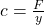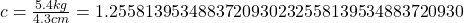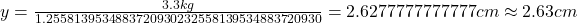## One end of a 7-cm-long spring is attached to the ceiling. When a 5.4 kg mass is hung from the other end, the spring is stretched by a length

Question

One end of a 7-cm-long spring is attached to the ceiling. When a 5.4 kg mass is hung from the other end, the spring is stretched by a length of 4.3 cm. How long is the spring when a 3.3 kg mass is suspended from it in cm?

in progress 0
2 months 2021-07-31T19:39:25+00:00 1 Answers 6 views 0

2.63 cm

Explanation:

Hooke’s law gives that the force F is equal to cy where c is spring constant and x is extension

Making c the subject of the formula thenSince F is gm but taking the given mass to be FBy substitution now considering F to be 3.3 kg